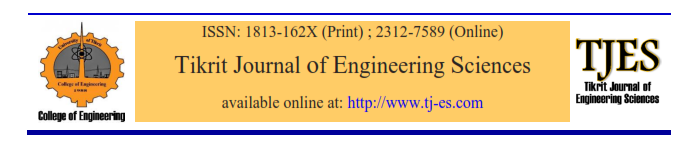# vol25no3pa7

TJES: Raheem AM. Total head evaluation using exact and finite element solutions of Laplace equation for seepage of water under sheet pile. Tikrit Journal of Engineering Sciences 2018; 25 (3): 40-46.
APA: Raheem, A. M. (2018). Total head evaluation using exact and finite element solutions of Laplace equation for seepage of water under sheet pile. Tikrit Journal of Engineering Sciences, 25(3), 40-46.

References

 Farid B, Naima B. Critical hydraulic head loss assessment for a circular sheet pile wall under axisymmetric seepage conditions. Studia Geotechnica et Mechanica 2011; 33 (4): 3–25.
 Terzaghi K. Theoretical soil mechanics. Wiley: New York; 1943.
 Suvasish M. Seepage analysis through foundation using FEM and Flownet. The 12th International Conference of International Association for Computer Methods and Advances in Geomechanics 2008; 1-6 October; Goa; India: 4175-4183.
 Hirani AN, Nakshatrala KB, Chaudhry JH. Numeri-cal method for Darcy flow derived using discrete exterior calculus. International Journal for Computational Methods in Engineering Science and Mechanics 2015; 16: 151–169.
 Liu GR, Dai KY, Nguyen TT. A smoothed finite element method for mechanics problems. Computati-onal Mechanics 2007; 39 (6): 859–877.

Tikrit Journal of Engineering Sciences (2018) 25(3) 40- 46Total Head Evaluation using Exact and Finite Element Solutions of Laplace Equation for Seepage of Water under Sheet Pile

 Aram Mohammed Raheem Dept. of Civil Eng., Kirkuk University, Iraq

Abstract

In this study, the strong and weak forms of Darcy flow equation has been derived. The final obtained equation is Laplace partial differential equation. For total head evaluation, Laplace equation is solved using exact solution depending on Fourier series and numerical solution depending on finite element method. A computer program has been developed in MATLAB program to solve the Laplace equation using both exact and finite element solutions and the seepage of water under sheet pile is taken as a case study. Different number of points and nodes has been selected for the two methods. It is shown that both exact and finite element solutions have a good match in distribution for the study area especially when the Fourier points are increased.Loading...Taking too long?Reload document
|Open in new tab